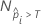# Methods and formulas for the lift chart for Fit Model and Discover Key Predictors with TreeNet® Classification

###### Note

The procedure for the points on the lift chart depends on the validation method. For a multinomial response variable, Minitab displays multiple charts that treat each class as the event in turn.

## Training set or no validation

For the chart for a training set, each point on the chart represents a fitted probability from the model. The highest event probability is the first point on the chart and appears leftmost. The other event probabilities are in decreasing order.

The points on the non-cumulative lift chart do not follow from the calculation of the points on the ROC curve chart. Instead, the y-coordinate of the non-cumulative lift chart is (non-cumulative true positive rate in percent / % of population at the x-coordinate). The calculation of the true positive rate is exactly the same as for the ROC curve chart.

The x-coordinate of the chart has the following form:

whereis the number of rows where the fitted probability is greater than the threshold and N is the total number of rows. For details on the thresholds, go to Methods and Formulas for the Receiver Operating Characteristic (ROC) curve chart for Fit Model and Discover Key Predictors with TreeNet® Classification.

## Separate test set

Use the same steps as the training set case but calculate the event probabilities from the cases for the test set.

## Test with k-fold cross-validation

Use the same steps as the training data set procedure, but calculate the event probabilities from the cases for the cross-validated data.

By using this site you agree to the use of cookies for analytics and personalized content.  Read our policy